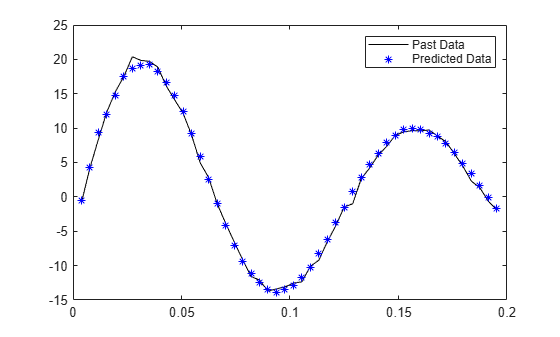## Forecast Output of Dynamic System

To forecast the output of a dynamic system, you first identify a model that fits past measured data from the system, and then forecast the future outputs of the identified model. Suppose that you have a set Y of N measurements of the output of a system (Y = {y1, y2, ...yN}). To forecast the outputs into the future:

1. Identify a model of the system using time series estimation commands such as `ar`, `arx`, `armax`, and `ssest`.

The software estimates the models by minimizing the squared sum of one-step ahead prediction errors. You can identify linear models such as AR, ARMA, and state-space models. You can also estimate nonlinear ARX and nonlinear grey-box models.

2. Validate the identified model using `predict` command.

The `predict` command predicts the output of an identified model over the time span of measured data (Yp = yp1, yp2, ...ypN). Use `predict` to determine if the predicted results Yp match the observed outputs Y for a desired prediction horizon. If the predictions are good over the time span of available data, use the model for forecasting.

3. Specify forecasting options such as how the software computes initial conditions of the measured data. To specify the options, use the `forecastOptions` option set.

4. Compute the output of the identified model until a future time horizon H, (yN+1, yN+2,..., yN+H) using the `forecast` command. Unlike the `predict` command, the `forecast` command performs prediction into the future, in a time range beyond the last instant of measured data.

The software computes the forecasted values by:

• Generating a predictor model using the identified model.

• Computing the final state of the predictor using measured (available) data.

• Simulating the identified model using the final state as initial conditions.

You can also forecast outputs for systems where measurable exogenous inputs u(t) influence the output observations. In this case, you first identify an input-output model using measured y(t) and u(t), and then use the `forecast` command.

### Forecast Time Series Data Using an ARMA Model

This example shows how to forecast time series data from a system using an ARMA model. Load the time series data that is to be forecasted.

```load iddata9 z9 past_data = z9.OutputData(1:50);```

Fit an ARMA model of order `[4 3]` to the measured data.

`sys = armax(past_data,[4 3]);`

Perform a 10-step ahead prediction to validate the model over the time-span of the measured data.

`yp = predict(sys,past_data,10);`

Plot the predicted response and the measured data.

```t = z9.SamplingInstants; t1 = t(1:50); plot(t1,past_data,'k',t1,yp,'*b') legend('Past Data','Predicted Data')```The plot shows that `sys` is a good prediction model that can be used for forecasting.

Specify zero initial conditions for the measured data.

`opt = forecastOptions('InitialCOndition','z');`

Forecast model output 100 steps beyond the estimation data.

```H = 100; yf = forecast(sys,past_data,H,opt);```

Plot the past and forecasted data.

```t2 = t(51:150)'; plot(t1,past_data,'k',t2,yf,'--r') legend('Past Data','Forecasted Data')```